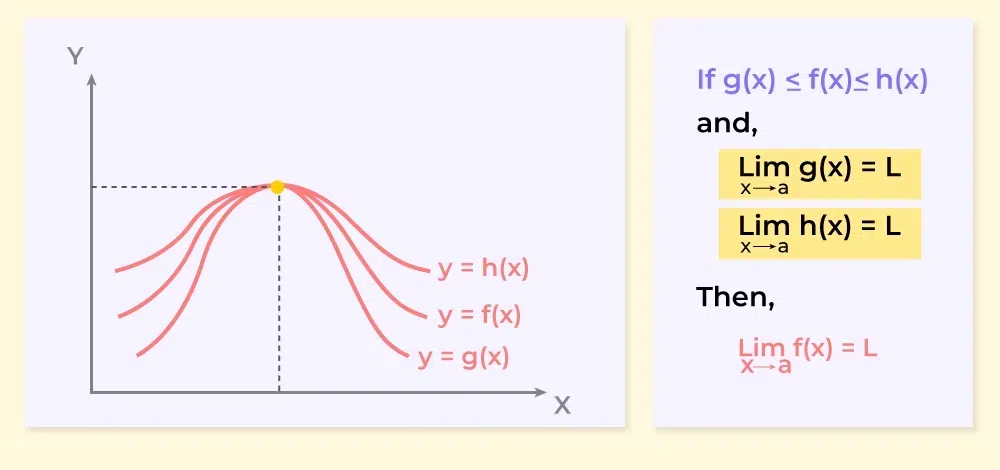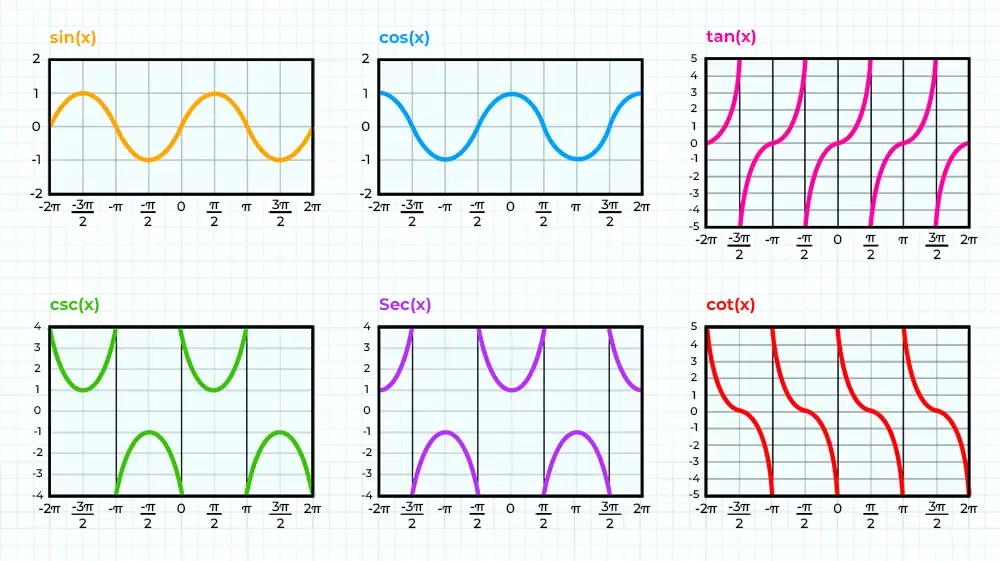GFG App
Open AppBrowser
Continue

We know that there are six trigonometric functions and the limit of trigonometric is the limit taken to each trigonometric function. We can easily find the limit of trigonometric functions and the limit of the trigonometric function may or may not exist depending upon the given function with the point of consideration.

For trigonometric functions and we can easily take the limit of all six trigonometric functions by replacing the function variable with the limit value. The limit of the trigonometric function depends on the domain, and range of the function. In this article, we will learn about the limit of all six trigonometric function, their examples and others in detail.

## Limits of Trigonometric Functions

The finite limit of trigonometric functions, i.e. the limit of the trigonometric function for the finite value of the x is discussed in the table below:

As seen from the table above it is evident that the finite limit of the trigonometric function results in a finite value except for some exceptions. We know that for sin x, and cos x their range is between -1 and 1 and so for the infinite limit their value oscillates between -1 and 1 finding their exact value is not possible and thus their lint at infinity is undefined. For x reaching positive or negative infinity, the limit of the trigonometric functions is discussed below.

### Function Limit of the function for ±∞

The infinite limit of the trigonometric function is discussed in the table below,

## Theorems of Limits of Trigonometric Functions

We have two theorems that are used in defining the limit of the trigonometric functions that are,

### Theorem 1

For any two real-valued functions f(x) and g(x) which are defined in the same domain and the relation between them is f(x) ≤ g(x). We can take the limit of these functions at x approaching a then,

limx⇢af(x) = f(a) and, limx⇢ag(x) = g(a)

If both limits exist then we can easily state that,

limx⇢af(x) ≤ limx⇢ag(x)

### Theorem 2 (Sandwich Theorem)

The theorem is used to calculate the limit of those functions whose limit cannot be calculated easily like (sin x/x at x = 0). The function g(x) is squeezed or sandwiched between two functions h(x) and g(x) in such a way that

f(x) ≤ g(x) ≤ h(x)

The graph of the above condition is shown below which represents the sandwich theorem limit.We can say h(x) is the upper limit of g(x) and f(x) is its lower limit at point a as can be observed in the graph above:

limx→a h(x) = L and limx→a f(x) = L

Where,

• a is the point at which the limit is calculated, and
• L is the value of the limit.

Then,

limx→a g(x) = L

Example: Given: g(x) = x2sin(1/x), Find: limx→0 g(x)

Solution:

We know,

-1≤ sin(1/x) ≤ 1 Under its domain

Multiplying with x2

-x2 ≤ x2sin(1/x) ≤ x2

Then let f(x) = -x2 and h(x) = x2

f(x) ≤ g(x) ≤ h(x)

Using sandwich theorem,

As limx→a h(x) = limx→a f(x) = L

Therefore,

limx→a g(x) = L

⇒ limx→0 f(x) = limx→0 -x2 = 0 and

⇒ limx→0 h(x) = limx→0 x2 = 0

Thus, limx→0 g(x) = 0

## Limits of Various Trigonometric Functions

As we know that we have six trigonometric functions, i.e.,

• Sine
• Cosine
• Tangent
• Secant
• Cosecant
• Cotangent

The limit of each function is discussed in detail in the article below.

### Limit of Sine Function

The function f(x) = sin(x) is a continuous function over its entire domain, with its domain consisting of all the real numbers. The range of this function is [-1, 1] as can be seen in the graph added below

So, if the limit of the sine function is calculated at any given real number it’s always defined and lies between [-1, 1].

Let, f(x) = sin(x)

limx→a f(x) = limx→a sin(x)

⇒ limx→a f(x) = sin(a),                    (where a is a real number)

Example: Find limx→π/2 sin(x)

Solution:

As we know sin(x) is defined at π/2

Therefore,

limx→π/2 sin(x) = sin(π/2) = 1

### Limit of Cosine Function

The function f(x) = cos(x) is a continuous function over its entire domain, with its domain consisting of all the real numbers.

The range of this function is [-1, 1] as can be seen in the graph added below.

So, if the limit of the cosine function is calculated at any given real number it’s always defined and lies between [-1, 1].

Let g(x) = cos(x)

limx→a g(x) = limx→a cos(x)

⇒ limx→a g(x) = cos(a), where a is a real number

Example: Find limx→π/2 cos(x)

Solution:

As we know cos(x) is continuous and defined at π/2

Therefore,

limx→π/2 cos(x) = cos(π/2) = 0

### Limit of Tangent Function

The function f(x) = tan(x) is defined at all real numbers except the values where cos(x) is equal to 0, that is, the values π/2 + πn for all integers n. Thus, its domain is all real numbers except π/2 + πn, n € Z.

The range of this function is (-∞, +c) as can be seen in the graph added below.

So, if the limit of the tangent function is calculated in its domain it is always defined and lies between(-∞, +∞).

f(x) = tan(x)

limx→a f(x) = limx→a  tan(x)

⇒ limx→a f(x) = tan(a)                 (where a belongs to real no. except π/2 + πn, n € Z)

Example: Find limx→π/2tan(x)

Solution:

As we can see in graph tan(x) is tending to +∞ as x→-π/2 and to -∞ as x→+π/2

So, the right-hand and left-hand limits of this function at π/2 are not same

Therefore,

limx→π/2 tan(x) = Not defined

### Limit of Cosec Function

The function f(x) = cosec(x) is defined at all real numbers except the values where sin(x) is equal to 0, that is, the values πn for all integers n. Thus, its domain is all real numbers except πn, n € Z.

The range of this function is (-∞,-1] U [1,+∞) as can be seen in the graph added below.

So, if the limit of the cosine function is calculated in its domain it is always defined and lies between its range.

Let f(x) = cosec(x)

limx→a f(x) = limx→a cosec(x)

⇒ limx→a f(x) = cosec(a)        (where a∈ R – nπ: n € Z)

Example: Find limx→π cosec(x)

Solution:

As we know sin(x) is 0 as x→π

So, 1/sin(x) i.e. cosec(x) at π cannot be defined

Therefore,

limx→π cosec(x) = Not defined

### Limit of Secant Function

The function f(x) = sec(x) is defined at all real numbers except the values where cos(x) is equal to 0, that is, the values π/2 + πn for all integers n. Thus, its domain is all real numbers except π/2 + πn, n € Z.

The range of this function is (-∞, -1] U [1, +∞) as can be seen in the graph added below.

So, if the limit of the sec function is calculated in its domain it is always defined and lies between its range.

Let f(x) = sec(x)

limx→a f(x) = limx→a sec(x)

⇒ limx→a f(x) = sec(a)          (where a∈ R – {nπ + π/2}: n € Z)

Example: Find limx→π/2 sec(x)

Solution:

As we know cos(x) is 0 as x→π/2

So, 1/cos(x) i.e. sec(x) at π/2 cannot be defined

Therefore,

limx→π/2 sec(x) = Not defined

### Limit of Cot Function

The function f(x) = cot(x) is defined at all real numbers except the values where tan(x) is equal to 0, that is, the values πn for all integers n. Thus, its domain is all real numbers except πn, n € Z.

The range of this function is (-∞, +∞) as can be seen in the graph added below.

So, if the limit of the cot function is calculated in its domain it is always defined and lies between its range.

Let f(x) = cot(x)

limx→a f(x) = limx→a cot(x)

⇒ limx→a f(x) = cot(a)               (where a∈ R – nπ: n € Z)

Example: Find limx→π cot(x)

Solution:

As we know tan(x) is 0   as x→π

So, 1/tan(x) i.e. cot(x) at π cannot be defined

Therefore,

limx→π cot(x) = Not defined

## Graph of Various Trigonometric Functions

The graph of various trigonometric functions is added in the following image:## Solved Examples on Limits of Trigonometric Functions

Example 1: Given: g(x) = cos(x)/x, Find: limx→∞ g(x)

Solution:

We know,

-1≤ cos(x)≤ 1 under its domain

Dividing by x,

-1/x ≤ cos(x)/x ≤ 1/x

Then let f(x) = -1/x and h(x) = 1/x

f(x) ≤ g(x) ≤ h(x)

Using sandwich theorem,

As limx→a h(x) = limx→a f(x) = L

Therefore,

limx→a g(x) = L

⇒ limx→∞ f(x) = limx→∞ -1/x = 0 and

⇒ limx→∞ h(x) = limx→∞ 1/x = 0

Therefore,

limx→∞ g(x) = 0

Example 2: Find limx→π/2 cot(x)

Solution:

As we know cot(x) is continuous and defined at π/2

Therefore,

limx→π/2 cot(x) = cot(π/2) = 0

Example 3: Find limx→π sec(x)

Solution:

As we know tan(x) is continuous and defined at π

Therefore,

limx→π cosec(x) = sec(π) = -1

Example 4: Find limx→π/2 cosec(x)

Solution:

As we know tan(x) is continuous and defined at π/2

Therefore,

limx→π/2 cosec(x) = cosec(π/2) = 1

Example 5: Find limx→πtan(x)

Solution:

As we know tan(x) is continuous and defined at π

Therefore,

limx→π tan(x) = tan(π) = 0

Example 6: Find limx→π cos(x)

Solution:

As we know cos(x) is continuous and defined at π

Therefore,

limx→π cos(x) = cos(π) = -1

Example 7: Find limx→πsin(x)

Solution:

As we know sin(x) is defined at π

Therefore,

limx→π sin(x) = sin(π) = 0

## FAQs on Limits of Trigonometric Functions

### Q1: What are trigonometric functions?

Trigonometric functions are the function that represents the relationship between the angles of the triangle with the sides of the triangle. They are also called circular functions as they repeat their value after some time in a circular manner.

### Q2: What are six basic fundamental trigonometric functions?

The six basic trigonometric functions are,

• Sine Function
• Cosine Function
• Tangent Function
• Secant Function
• Cosecant Function
• Cotangent Function

### Q3: Is the limit of the trigonometric function exist at infinity?

As we know that trigonometric functions are cyclic functions and generally their values oscillate between two values. So their values at infinity do not exist.